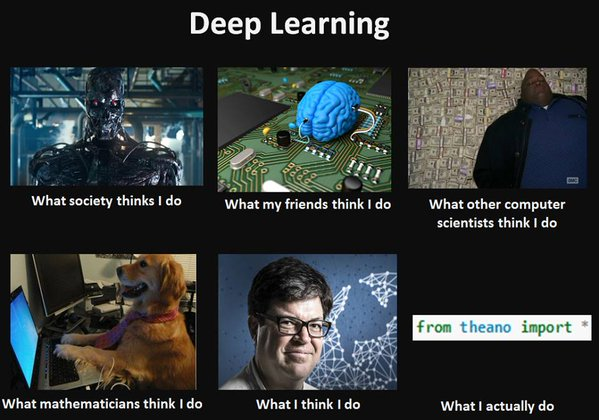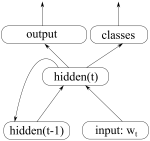# Month: January 2016

## Theano Tutorial

This is an introductory tutorial on using Theano, the Python library. I’m going to start from scratch and assume no previous knowledge of Theano. However, understanding how neural networks work will be useful when getting to the code examples towards the end.

The plan for the tutorial is as follows:

1. Give a basic introduction to Theano and explain the important concepts.
2. Go over the main operations that we have available in Theano.
3. Look at working code examples.

I recently gave this tutorial as a talk in University of Cambridge and it turned out to be way more popular than expected. In order to give more people access to the material, I’m now writing it up as a blog post.

I do not claim to know everything about Theano, and I constantly learn new things myself. If you find any errors or have suggestions on how to improve this tutorial, do let me know.

The code examples can be found in the Github repository: https://github.com/marekrei/theano-tutorial

## 1. What is Theano?Theano is a Python library for efficiently handling mathematical expressions involving multi-dimensional arrays (also known as tensors). It is a common choice for implementing neural network models. Theano has been developed in University of Montreal, in a group led by Yoshua Bengio, since 2008.

Some of the features include:

• automatic differentiation – you only have to implement the forward (prediction) part of the model, and Theano will automatically figure out how to calculate the gradients at various points, allowing you to perform gradient descent for model training.
• transparent use of a GPU – you can write the same code and run it either on CPU or GPU. More specifically, Theano will figure out which parts of the computation should be moved to the GPU.
• speed and stability optimisations – Theano will internally reorganise and optimise your computations, in order to make them run faster and be more numerically stable. It will also try to compile some operations into C code, in order to speed up the computation.

## Online Representation Learning in Recurrent Neural Language Models

In a basic neural language model, we optimise a fixed set of parameters based on a training corpus, and predictions on an unseen test set are a direct function of these parameters. What if instead of a static model we constantly measured the types of errors the model is making and adjust the parameters accordingly? It would potentially be more closer to how humans operate, constantly making small adjustments in their decisions based on feedback.

The necessary information is already available – language models use the previous word in the sequence as context, which means they know the correct answer for the previous time step (or at least need to assume they know). We can use this to calculate error derivatives at each time step and update parameters even during testing. This sounds like it would require loads of extra computation at test time, but by updating only a small part of the model we can actually get better results with faster execution and fewer parameter.

This post is a summary of my EMNLP 2015 paper “Online Representation Learning in Recurrent Neural Language Models“.

## RNNLM

First a short description of the RNN language model that I use as a baseline. It follows the implementation by Mikolov et al. (2011) in the RNNLM Toolkit.The previous word goes into the network as a 1-hot vector which is then multiplied with a weight matrix, giving us a corresponding word embedding. This, together with the previous hidden state, act as input to the current hidden state of the network:

$$hidden_t = \sigma(E \cdot input_t + W_h \cdot hidden_{t-1})$$